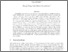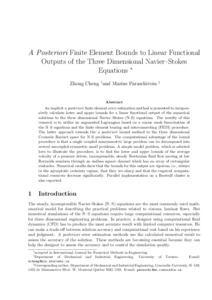Title:

# A posteriori finite element bounds to linear functional outputs of the three-dimensional Navier-Stokes equations

Cheng, Zhong and Paraschivoiu, Marius (2004) A posteriori finite element bounds to linear functional outputs of the three-dimensional Navier-Stokes equations. International Journal for Numerical Methods in Engineering, 61 (11). pp. 1835-1859. ISSN 0029-5981Preview
Text (pre-print ) (application/pdf)
CP2004.pdf - Submitted Version
362kB

Official URL: http://dx.doi.org/10.1002/nme.1132

## Abstract

An implicit a posteriori finite element error estimation method is presented to inexpensively calculate lower and upper bounds for a linear functional output of the numerical solutions to the three-dimensional Navier-Stokes (N-S) equations. The novelty of this research is to utilize an augmented Lagrangian based on a coarse mesh linearization of the N-S equations and the finite element tearing and interconnecting (FETI) procedure. The latter approach extends the a posteriori bound method to the three-dimensional Crouzeix-Raviart space for N-S problems. The computational advantage of the bound procedure is that a single coupled non-symmetric large problem can be decomposed into several uncoupled symmetric small problems. A simple model problem, which is selected here to illustrate the procedure, is to find the lower and upper bounds of the average velocity of a pressure driven, incompressible, steady Newtonian fluid flow moving at low Reynolds numbers through an endless square channel which has an array of rectangular obstacles. Numerical results show that the bounds for this output are rigorous, i.e. always in the asymptotic certainty regime, that they are sharp and that the required computational resources decrease significantly. Parallel implementation on a Beowulf cluster is also reported.

Divisions: Concordia University > Gina Cody School of Engineering and Computer Science > Mechanical and Industrial Engineering Article No Cheng, Zhong and Paraschivoiu, Marius International Journal for Numerical Methods in Engineering October 2004 Natural Sciences and Engineering Research Council of Canada 10.1002/nme.1132 output bounds • Crouzeix-Raviart elements • low Reynolds flows 6757 ANDREA MURRAY 05 Jul 2010 17:17 18 Jan 2018 17:29

## References:

1. Ainsworth M, Oden JT. A unified approach to a posteriori error estimation using element residual methods. Numerische Mathematik 1993; 65: 23-50.

2. Verfürth R. A posteriori error estimators for the Stokes equations. Numerische Mathematik 1989; 55: 309-325.

3. Bank RE, Welfert BD. A posteriori error estimates for the Stokes problem. SIAM Journal on Numerical Analysis 1991; 28: 591-623.

4. Maday Y, Patera AT, Peraire J. A general formulation for a posteriori bounds for output functionals of partial differential equations; application to the eigenvalue problem. Comptes Rendus des seances de l'Academie des Sciences Paris Serie I 1999; 328: 828-836.

5. Paraschivoiu M. A posteriori finite element bounds for linear-functional outputs of coercive partial differential equations and of the Stokes problems. Ph.D. Thesis, Department of Mechanical Engineering, Massachusetts Institute of Technology, October 1997.

6. Paraschivoiu M, Peraire J, Patera AT. A posteriori finite element bounds for linear-functional outputs of elliptic partial differential equations. Computer Methods in Applied Mechanics and Engineering 1997; 150: 289-312.

7. Machiels L, Peraire J, Patera AT. A posteriori finite element output bounds for the incompressible Navier-Stokes equations: application to a natural convection problem. Journal of Computational Physics 2001; 172: 401-425.

8. Cheng Z, Paraschivoiu M. Parallel computions of finite element output bounds for conjugate heat transfer. Finite Element in Analysis and Design 2003; 39/7: 581-597.

9. Ladeveze P, Leguillon D. Error esimation procedures in the finite element method and applications. SIAM Journal on Numerical Analysis 1983; 20: 485-509.

10. Farhat C, Roux F-X. An unconventional domain decomposition method for efficient parallel solution of large-scale finite element systems. SIAM Journal on Scientific and Statistical Computing 1992; 13(1): 379-396.

11. Paraschivoiu M. A posteriori finite element output bounds in three space dimensions using the FETI method. Computer Methods in Applied Mechanics and Engineering 2001; 190(49-50): 6629-6640.

12. Maday Y, Patera AT. Numerical analysis of a posteriori finite element bounds for linear-functional outputs. Mathematical Models & Methods in Applied Science 2000; 10(5): 785-799.

13. Munson BR, Young DF, Okiishi TH. Fundamentals of Fluid Mechanics. Wiley: New York, 1990.

14. Quarteroni A, Valli A. Numerical Approximation of Partial Differential Equation. Springer: Berlin, 1994.

15. Temam R. Navier Stokes Equations: Theory and Numerical Analysis. North-Holland Publishing: Amsterdam, 1979.

16. Gunzberger MD. Finite Element Methods for Viscous Incompressible Flows, A Guide to Theory, Practice, and Algorithms. Academic Press: San Diego, 1989.

17. Oden JT, Wu W, Ainsworth M. An a posteriori error estimate for finite element approximations of the Navier-Stokes equations. TICOM Report 93-05, 1993.

18. Zienkiewicz OC, Liu YC, Huang GC. Error estimates and convergence rates for various incompressible elements. International Journal for Numerical Methods in Engineering 1989; 28: 2191-2202.

19. Cheng Z. A posteriori finite element bounds: application to outputs of the three dimensional Navier-Stokes equations. Ph.D. Thesis, Graduate Department of Mechanical and Industrial Engineering, University of Toronto, April 2003.

20. Farhat C, Chen P-S, Risler F, Roux F-X. A unified framework for accelerating the convergence of iterative substructuring methods with Lagrange multipliers. International Journal for Numerical Methods in Engineering 1998; 42: 257-288.

21. Farhat C, Pierson K, Lesoinne M. The second generation FETI methods and their application to parallel solution of the large-scale linear and geometrically non-linear structural analysis problems. Computer Methods in Applied Mechanics and Engineering 2000; 184: 333-374.

22 Farhat C, Roux F-X. A method of finite tearing and interconnecting and its parallel solution algorithm. Computer Methods in Applied Mechanics and Engineering 1991; 32: 1205-1227.
All items in Spectrum are protected by copyright, with all rights reserved. The use of items is governed by Spectrum's terms of access.

Repository Staff Only: item control pageResearch related to the current document (at the CORE website)
Back to top